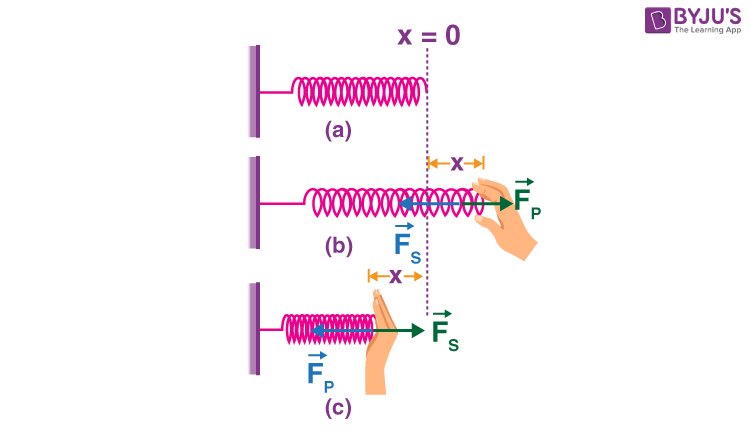Jet Set Go! All about Aeroplanes Jet Set Go! All about Aeroplanes

# Potential Energy Of A Spring

When we compress or extend a stretched spring, we experience a force equal to that applied by us in the opposite direction. Have you wondered why this happens? When a spring deviates from its mean position, it tends to restore its equilibrium by exerting a force equal and opposite to the external force. But in which way can this force be helpful to us? Can we harness it in any form? We have seen the application of spring force in bicycle carriers and launching devices where the energy gained by disturbing the equilibrium of the spring is utilized as its potential energy and converted to other forms. In this section, we will learn more about this stored energy. In this session, let us learn about work done by spring.

## Work Done By SpringLet us consider a spring fixed to a rigid wall, as shown in the figure above. The spring is assumed to be ideal and considered as massless. In figure (a), the spring is at the equilibrium position. In figure (b), the spring is stretched such that it gets displaced by a value x from its equilibrium position and in figure (c), it is compressed such that it gets displaced by a value –x from its equilibrium position.

In an ideal spring, the spring force is proportional to its ends’ displacement (x) from the equilibrium position. So, according to Hooke’s Law,

Fs = -kx

Where Fs is the spring force, x is the displacement from the equilibrium position, and k is the spring constant. The spring constant is the characteristic property of the spring. It depends upon the construction material and is measured in the units of N m-1.

As shown in fig (b) above, we have pulled the spring such that resultant displacement equal to xm is measured. The work done by the spring is given by,

$$\begin{array}{l}W_{s}=\int_{0}^{x}F_{s}dx=-\int_{0}^{x}kxdx=-\frac{k(x)^{2}}{2}\end{array}$$

Here the work done by the external pulling force Fp is given by

$$\begin{array}{l}F_{p}=\frac{K(x)^{2}}{2}\end{array}$$

which is positive since it overcomes the spring force.

The same is observed for a spring being compressed by a distance x.

If we move the spring from an initial displacement Xi to a final displacement Xf, the work done by the spring force is given as,

$$\begin{array}{l}W_{s}=-\int_{X_{i}}^{X_{f}}kxdx =\frac{K(X_{i})^{2}}{2}-\frac{K(X_{f})^{2}}{2}\end{array}$$

The potential energy V(x) of the spring is considered to be zero when the spring is at the equilibrium position. When it is extended to a displacement X, the ends are stationary; hence the kinetic energy is zero. Thus, the potential energy is equal to the total external work done on the system.

Hence,

$$\begin{array}{l}W_{ext}=W_{p}=V(x)=\frac{K(X)^{2}}{2}\end{array}$$

## Frequently Asked Questions – FAQs

### What is the formula to find potential energy?

W = m×g×h = mgh
Where,
• m is the mass in kilograms
• g is the acceleration due to gravity
• h is the height in meters
• ### State Hooke’s law

Hooke’s law states that the strain of the material is proportional to the applied stress within the elastic limit of that material.

True.

### Give the expression for the work done by spring

The expression for the work done by spring is given by:

$$\begin{array}{l}W_{ext}=W_{p}=V(x)=\frac{K(X)^{2}}{2}\end{array}$$

### Define spring constant.

The spring constant is the characteristic property of the spring. It depends upon the construction material and is measured in the units of N m-1.Hope you learned the derivation of work done by spring. Stay tuned with BYJU’S to learn more about the potential energy of spring and other related topics and much more.

Test Your Knowledge On Potential Energy Spring!